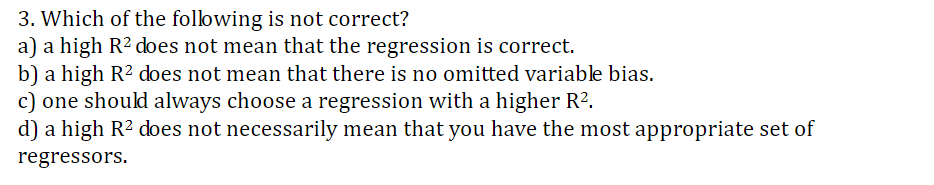# 3. Which of the following is not correct?a) a high R2 does not mean that the regression is correctb) a high R2 does not mean that there is no omitted variable biasc) one should always choose a regression with a higher R2.d) a high R2 does not necessarily mean that you have the most appropriate set ofregressors

Question
1 viewshelp_outlineImage Transcriptionclose3. Which of the following is not correct? a) a high R2 does not mean that the regression is correct b) a high R2 does not mean that there is no omitted variable bias c) one should always choose a regression with a higher R2. d) a high R2 does not necessarily mean that you have the most appropriate set of regressors fullscreen
check_circle

Step 1

The correct option is: (c) One should always choose a regression with a higher R2.

The R2 value tells the percentage of variation in the dependent variable that is explained by the independent variable.

The higher R2 value does not mean that ‘there are most appropriate...

### Want to see the full answer?

See Solution

#### Want to see this answer and more?

Solutions are written by subject experts who are available 24/7. Questions are typically answered within 1 hour.*

See Solution
*Response times may vary by subject and question.
Tagged in

### Other